## What is a 3-Statement Model?

A 3-statement model forecasts a company’s income statement, balance sheet, and cash flow statement by linking them. The aim of a financial model is to predict a company’s profitability, financial position, and cash generation; building a 3-statement model improves the accuracy of forecasting because a change in one financial statement will result in adjustments to the others. These adjustments act as a check on the validity of the assumptions and forecasts.

3 statement models are built in Excel and typically the income statement is created first, followed by the balance sheet and then the cash flow statement. The cash flow statement helps forecast cash and short-term borrowings; this is an important step in ensuring that the model links correctly. The final step is to calculate interest expenses and include them in the income statement.

## Key Learning Points

• 3-statement modeling links the forecast income statement, balance sheet, and cash flow statement.
• A change in one financial statement will flow through to the others, acting as a check on the validity of the forecasts.
• A 3-statement model usually starts with the income statement, then the balance sheet, and finally the cash flow statement.
• The cash flow statement helps forecast cash and short-term borrowings and is an important step in linking the three statements.
• Short-term borrowings are often referred to as a “revolver” – an abbreviation for a revolving credit facility.

## 9 Steps in Building a 3-Statement Model

The nine steps in building a 3-statement model are: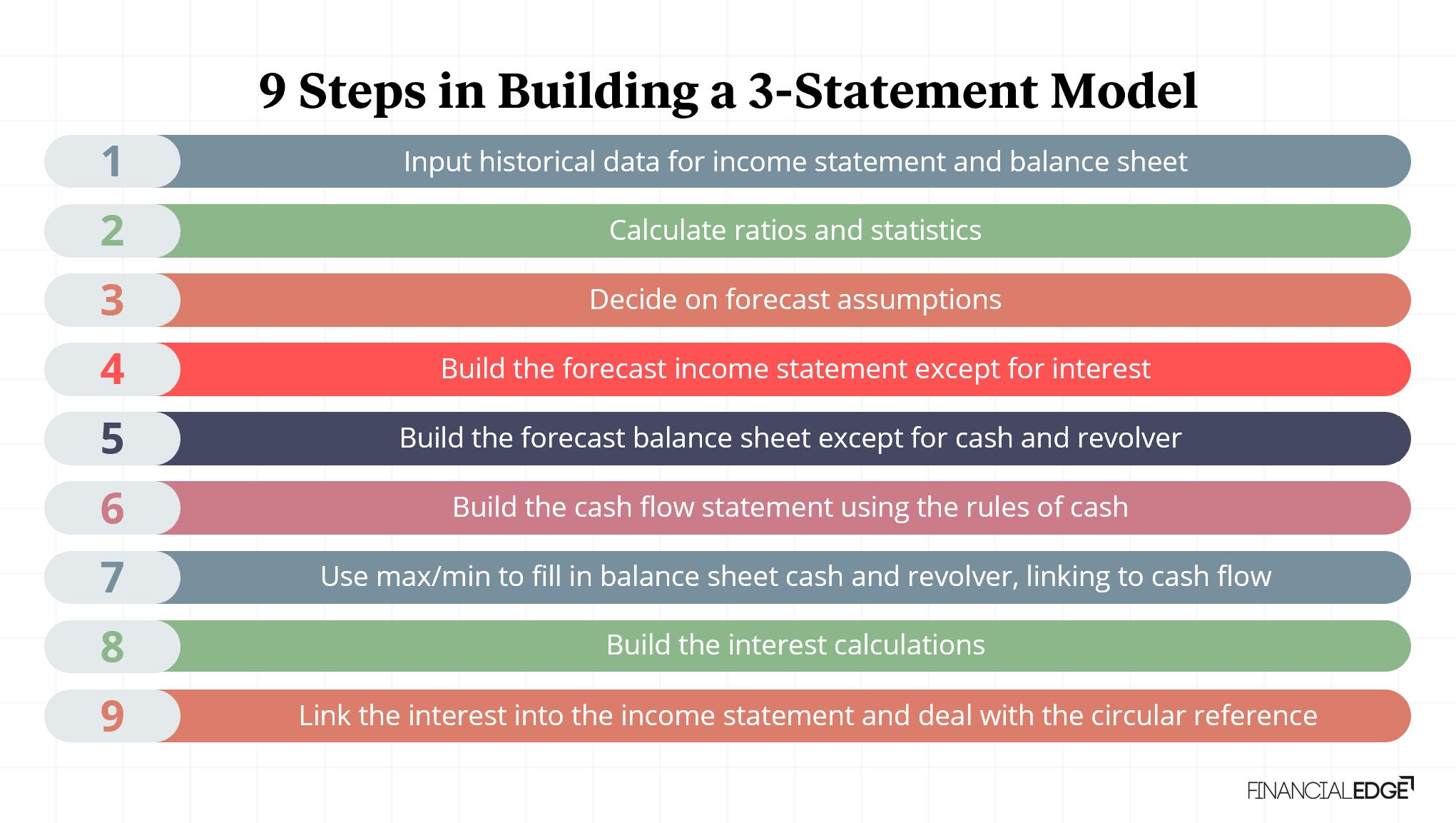1. Input the historical data: Start by inputting historical data (referred to as “actuals”) for the income statement and balance sheet.
2. Calculate ratios and statistics: These are calculated using the historical data to help understand historical performance and business drivers.
3. Decide on forecast assumptions: Use the ratios and statistics to create forecast assumptions. For example, understanding historical performance could help us predict that sales will increase by 5% each year during the forecast period.
4. Build forecast income statement except for interest: Forecast each income statement using the assumptions – this will include sales increasing by 5% each year. However, we can’t forecast interest income and expense at this point as the cash and debt balances haven’t yet been forecast.
5. Build the forecast balance sheet except for cash and revolver: Forecast each balance sheet item using the assumptions. However, we can’t forecast cash and the revolver at this point as this relies on the forecast cash flow statement.
6. Build the cash flow statement using the rules of cash: Forecast the cash flow statement using the forecast income statement and balance sheet and the rules of cash. We calculate ‘cash net of revolver’ at the bottom of the cash flow statement – effectively treating a revolver as a negative cash balance.
7. Use max/min to fill in balance sheet cash and revolver: A positive ‘cash net of revolver’ balance is included in the balance sheet as cash (with a zero-revolver balance) while a negative ‘cash net of revolver’ balance is included in the balance sheet as a revolver (with a zero cash balance). We use the Excel max and min function for this as follows: a) Cash balance = MAX (0, ending cash net of revolver) b) Revolver balance = -MIN (0, ending cash net of revolver)
8.  Build the interest calculations: Interest income is calculated using the forecast cash balance and interest expense is calculated using the forecast revolver and long-term debt balances. Interest income and expense are typically calculated using the average of opening and closing balances.
9. Link interest into the income statement and deal with circular references: Interest can now be linked into the income statement and any circular references that arise will need to be resolved. The interest will lead to some changes in the net income, which will, in turn, affect the cash flow statement and cash on the balance sheet. Assuming we built our model correctly, this should all balance.

## Example – Forecasting the Cash Flow Statement, Cash, and Revolver

Given below are extracts of a model for The Hershey Company. We will use these to show how to build a forecast cash flow statement and to forecast cash and the revolver. We will build our calculations only in the first forecast year.

Forecast net income (excluding interest) for Year 1 is \$1,793.1m. A part-complete forecast balance sheet and calculations are provided below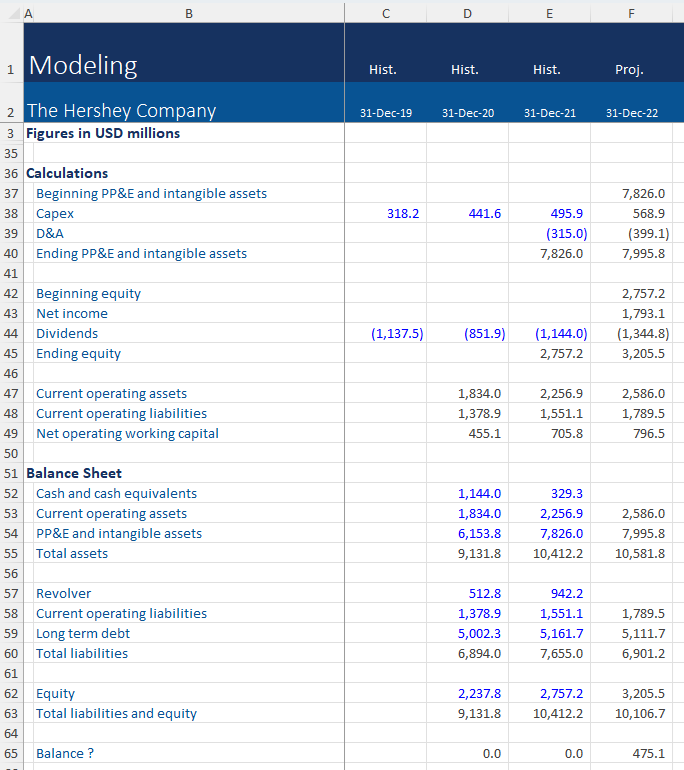At this stage, it’s important to note that the balance sheet doesn’t balance because we have not yet forecasted cash or the revolver.

Our rules of cash are:

• An increase in assets results in a cash outflow
• A decrease in assets results in a cash inflow
• An increase in liabilities or equity results in a cash inflow
• A decrease in liabilities or equity results in a cash outflow

Applying these rules to the forecast balance sheet allows us to build the forecast cash flow statement as follows: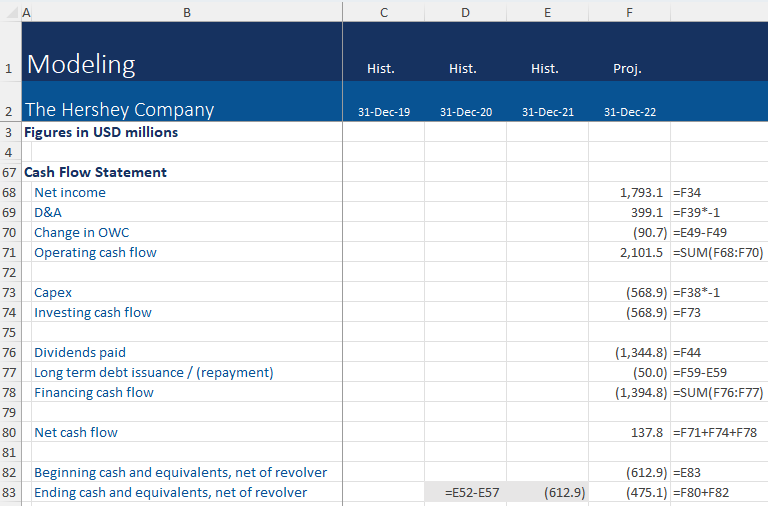The balance at the bottom of the cash flow statement is (\$475.1m), which is effectively a negative cash balance. This is a liability and needs to be included as a revolver balance of \$475.1m in our forecasts, while the forecast cash balance will be zero.

We use the MAX and MIN functions to forecast the cash and revolver balance as shown below – note that we include a minus sign before the MIN function to ensure that the revolver balance is shown as a positive number in our model.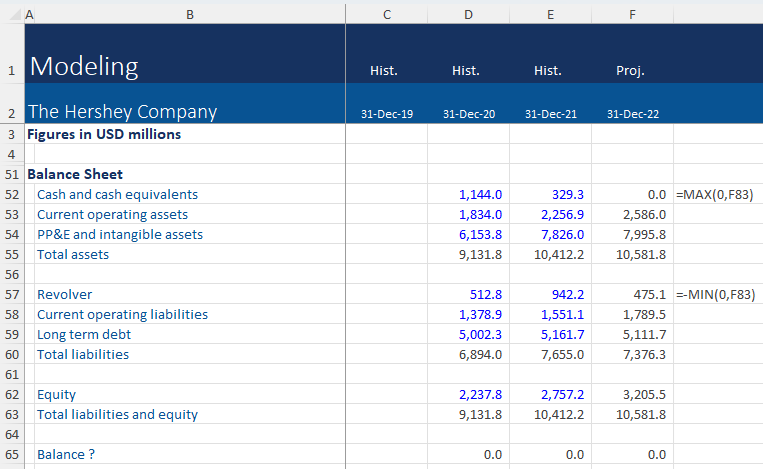At this point the balance sheet should now be balanced – this is an important way to check the integrity and accuracy of a 3-statement model.

The final steps to complete the model are to build the interest calculations using average cash and debt balances and then to include interest in the income statement.

Before sharing your model with your colleagues, it is important to do a final check of the following: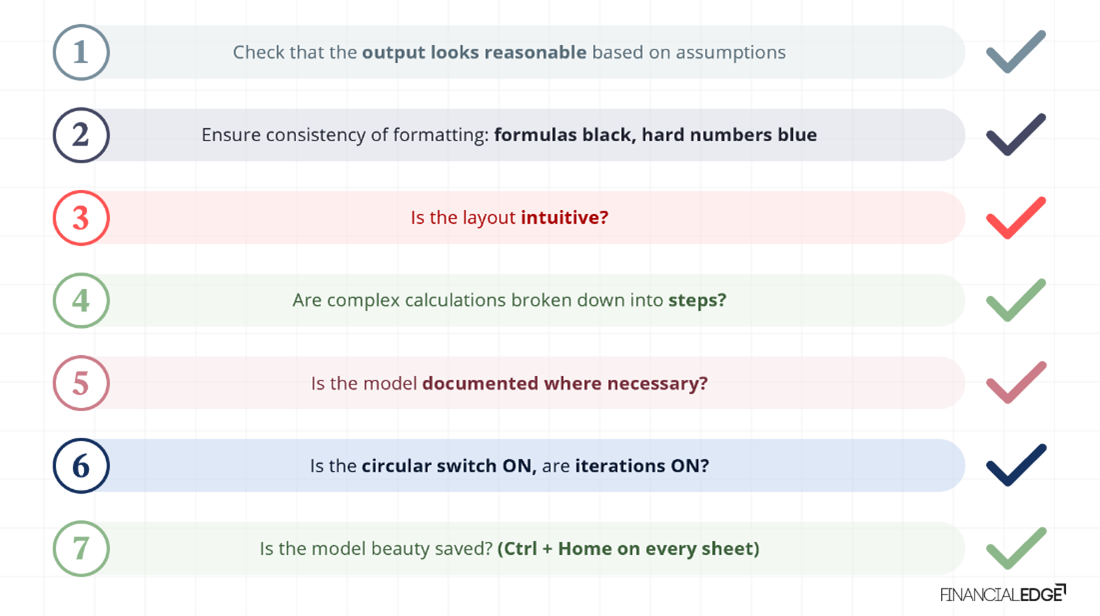Download the accompanying Excel exercise sheets to practice 3-statement modeling and to access the completed model for the Hershey Company. Take our online financial modeling course & learn how to build financial models from Wall Street instructors.

## Conclusion

A 3-statement model forecasts a company’s income statement, balance sheet, and cash flow statement by linking them. A change in one financial statement will flow through to the others, acting as a check on the validity of the forecasts. The model usually starts with the income statement, then the balance sheet, and finally the cash flow statement. The cash flow statement helps forecast cash and short-term borrowings and is an important step in linking the three statements. Short-term borrowings are often referred to as a “revolver” which is an abbreviation for a revolving credit facility.